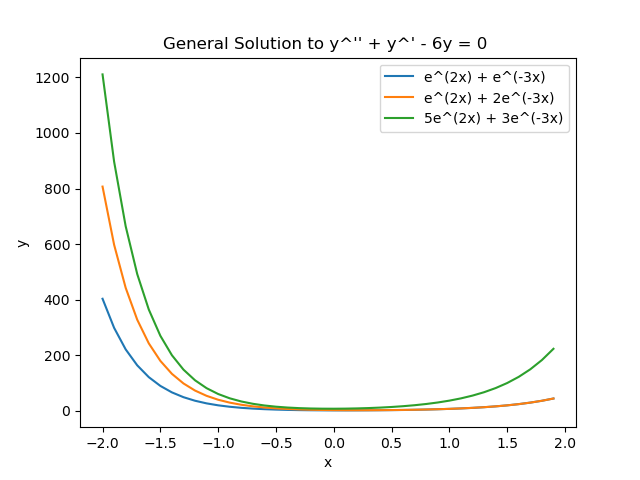# Solutions to Differential Equations

A solution to a differential equation is a function that satisfies the DE. The solution must be continueously differentiable on an interval, I. You can check the solution by taking the appropriate derivatives and confirming the LHS = RHS.

Confirm that $$y(t)=e^{-2t}$$ is a solution to $$y^{''}+y^{'}-2y=0$$.

There are different type of solutions discussed below:

 Type Description Example Trival Solution The solution $$y=0$$ is a trivial solution. $$y=0$$ General/Family Solution Solution with constants that can be solved given the initial value. Sometimes refered to as a familiy of solution as any constant will solve the DE. $$y=c_1 e^{3t}$$ Particular Solution Solution to a particular set of initial values. $$y=\frac{1}{6} e^{3t}$$ Implicit Solution Solution that is not defined explicitly as $$y = y(x)$$. $$\sin x + \cos y = 1$$

Show that $$x^2 + y^2 = 1$$ is a solution to the DE $$(y^{'})^2 + 1 = -yy^{''}$$.

## Initial Value Problems

To solve for the particular solution to a DE, often an initial value is provided to allow us to solve for the constants. For a DE of order n, initial values must be provided up to the n-1 derivative. For exmaple, for DE such as $$\cfrac{d^3y}{dx^3}=f(x,y,dy / dx, d^2y / dx^2)$$ must be subject to initial conditions $$y(x_0) = y_0, dy / dx (x_0) = y_1, d^2y / dx^2 (x_0) = y_2$$.

Consider the general solution $$y = c_1 e^{2x} + c_2 e^{-3x}$$ to the DE $$y^{''}+y^{'}-6y=0$$. We can show that this is indeed a solution:

$$y^{''}+y^{'}-6y=0$$

$$(4c_1 e^{2x} + 9c_2 e^{-3x})+ (2c_1 e^{2x} -3c_2 e^{-3x}) - 6(c_1 e^{2x} + c_2 e^{-3x})= 0$$

$$0 = 0$$

This is true for any value of $$c_1, c_2$$. Shown graphically below:Now we can find the particular solution given the contraints $$y(0) = 1, y^{'} (0) = 0$$

$$y = c_1 e^{2x} + c_2 e^{-3x}$$

$$y(0) = c_1 e^{2(0)} + c_2 e^{-3(0)}$$

$$1 = c_1 + c_2$$

$$y{'} = 2c_1 e^{2x} -3c_2 e^{-3x}$$

$$y{'}(0) = 2c_1 e^{2(0)} -3c_2 e^{-3(0)}$$

$$0 = 2c_1 - 3c_2$$

Solving the two equations for two unknowns yields:

$$c_1 = \frac{3}{5}, c_2 = \frac{2}{5}$$

$$y = \frac{3}{5} e^{2x} + \frac{2}{5} e^{-3x}$$